## LetsPlayMaths.Com

WELCOME TO THE WORLD OF MATHEMATICS

# Class 5 Arithmetic Operation

Addition of Large Numbers

Subtraction of Large Numbers

Multiplication of Large Numbers

Division of Large Numbers

Order of Operation

Order of Operation Using Brackets

Arithmetic Operation Test

Arithmetic Operation Worksheet

Answer Sheet

## Addition of Large Numbers

We have learnt addition of smaller numbers in our previous classes. The process for adding large numbers is the same as that for small numbers. To add large numbers, arrange the digits in columns according to the place value of digits. Then keep adding digits starting from ones place to the largest place. Let us have a look at some examples.

O - Ones

T - Tens

H - Hundred

Th - Thousands

TTh - Ten Thousand

L- Lakh

TL - Ten Lakh

C - Crores

TC - Ten Crores

Examples 1. Add 53456341 and 22607265.

Solution. Arrange the numbers in tabular format as shown below.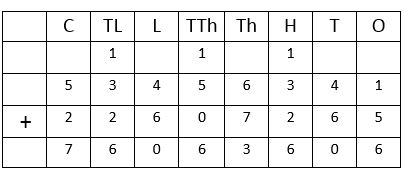So, the answer is 76063606.

Example 2. Add 243576125 and 650726578.

Solution. Arrange the numbers in tabular format as shown below.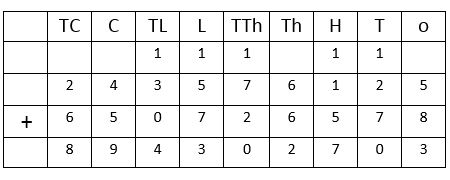So, the answer is 894302703.

## Subtraction of Large Numbers

We have learnt subtraction of smaller numbers in our previous classes. The process for subtracting large numbers is the same as that for small numbers. Let us have a look at some examples.

Example 1. Subtract 22607265 from 53456341.

Solution. Arrange the numbers in tabular format as shown below.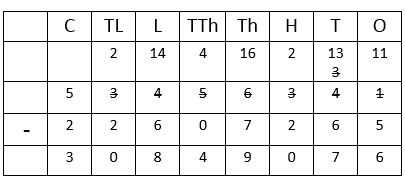So, the answer is 30849076.

Example 2. Subtract 243576125 from 650726578.

Solution. Arrange the numbers in tabular format as shown below.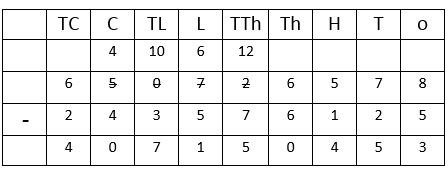So, the answer is 407150453.

## Multiplication of Large Numbers

In our previous classes, we have learnt multiplication of large number by a small number. Here we will use the same
technique to multiply large numbers by another large number. Let us have a look at some examples.

Example 1. Multiply 76432 by 5245.

Solution.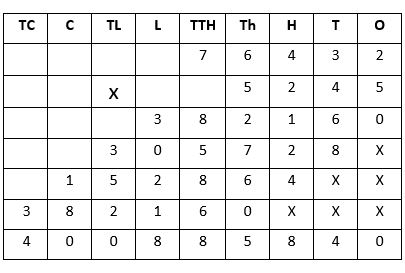So, the answer is 400885840.

Example 2. Multiply 575235 by 1250.

Solution.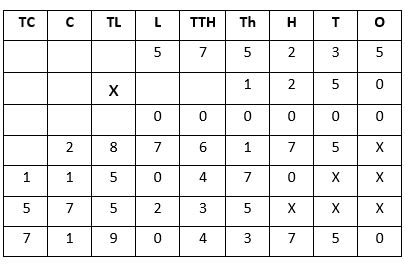So, the answer is 719043750.

## Division of Large Numbers

We have learnt divisions of small numbers in our earlier classes, we will use same methods to divide larger numbers.
Let’s have a look at some examples.

Example 1. Divide 12743775 by 5225.

Solution.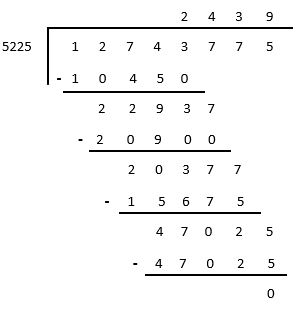So, the answer is 2439.

## Order of Operation

When all the operations are present in a problem, we first divide, then multiply, then addition and finally subtraction
happen. Let’s have a look at some examples.

Example 1. 9 + 15 X 3 – 12 + 5 – 6 ÷ 2 = ?

Solution. As per the rule, we must perform the division first and simplify the above given equation.

9 + 15 X 3 – 12 + 5 – 3

Then, we must perform the multiplication.

9 + 45 – 12 + 5 – 3

Arrange the ‘+’ numbers and ‘-‘ numbers separately. Then, we must perform the addition.

9 + 45 + 5 – 12 – 3 = 59 – 15

At last, we must perform the subtraction.

59 - 15 = 44

So, the answer is 44.

Example 2. Simplify 20 + 8 X 5 – 25 + 48 ÷ 8 + 27 ÷ 9

Solution. 20 + 8 X 5 – 25 + 48 ÷ 8 + 27 ÷ 9

Perform the division operation

20 + 8 X 5 – 25 + 6 + 3

Then, perform the multiplication

20 + 40 – 25 + 6 + 3

Arrange the ‘+’ numbers and ‘-‘ numbers separately.

20 + 40 + 6 + 3 – 25

Then, perform the addition

69 – 25

Finally, perform the subtraction

69 -25 = 44

So, the answer is 44.

## Order of Operation Using Brackets

If an equation is having brackets in it, then we must perform the operations inside the bracket first. After completion of bracket’s operation, then we must perform the division, then multiplication, then addition and at last the subtraction. We can memorize the sequence of operation by using this acronym (BODMAS).

B   -   Bracket

O   -   Of (Multiply the two numbers containing of)

D   -   Division

M   -   Multiplication

A   -   Addition

S   -   Subtraction

Let’s have a look at some examples.

Example 1. Simplify 45 + (6X2 – 1) + (18 ÷ 3 + 4) – 20

Solution. 45 + (6X2 – 1) + (18 ÷ 3 + 4) – 20

= 45 + (12 – 1) + (6 + 4) – 20

= 45 + 11 + 10 – 20

= 66 – 20 = 46

Example 2. Simplify 25 + {(18 ÷ 2 + 1) – 5} ÷ 5 of 1 + 3 x 5

Solution. 25 + {(18 ÷ 2 + 1) – 5} ÷ 5 of 1 + 3 x 5

= 25 + {(9 + 1) – 5} ÷ 5 of 1 + 3 X 5

= 25 + {10 – 5} ÷ 5 of 1 + 3 X 5

= 25 + 5 ÷ 5 + 3 X 5

= 25 + 1 + 3 X 5

= 25 + 1 + 15

= 41

## Class-5 Arithmetic Operation Test

Arithmetic Operation Test - 1

Arithmetic Operation Test - 2

## Class-5 Arithmetic Operation Worksheet

Arithmetic Operation Worksheet - 1

Arithmetic Operation Worksheet - 2

Arithmetic Operation Worksheet - 3

Arithmetic Operation Worksheet - 4

Arithmetic Operation Worksheet - 5

## Answer Sheet

Arithmetic-Operation-AnswerDownload the pdf

Copyright © 2021 LetsPlayMaths.com. All Rights Reserved.